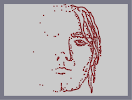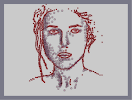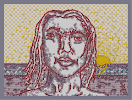### YEAH! PICK THAT NOSE!Hover over the thumbnail for a full-size version.

Author espada777777 author:espada777777 espada nose pick rated that 2010-01-24 2010-01-24 4 by 58 people. \$YEAH! PICK THAT NOSE!#espada777777#pick#00000000000000000000000000000000000000000000000000000000000000000000000000000000000000000000000000000000000000000000000000000000000000000000000000000000000000000000000000000000000000000000000000000000000000000000000000000000000000000000000000000000000000000000000000000000000000000000000000000000000000000000000000000000000000000000000000000000000000000000000000000000000000000000000000000000000000000000000000000000000000000000000000000000000000000000000000000000000000000000000000000000000000000000000000000000000000000000000000000000000000000000000000000000000000000000000000000000000000000000000000000000000000000000000000000000000000000000000000000000000000000000000000000000000000000000000000000000000000000|10^268,287!10^264,290!10^263,291!10^261,296!10^259,299!10^258,303!10^257,305!10^257,307!10^256,309!10^255,312!10^253,317!10^252,320!10^250,327!10^249,330!10^249,334!10^248,338!10^245,340!10^245,342!10^243,345!10^242,349!10^241,353!10^240,357!10^239,362!10^237,368!10^234,373!10^233,376!10^231,381!10^231,383!10^229,386!10^226,390!10^223,393!10^222,396!10^219,400!10^218,404!10^215,407!10^212,413!10^211,415!10^209,418!10^207,421!10^206,423!10^204,427!10^203,429!10^201,432!10^200,434!10^198,437!10^194,440!10^192,443!10^191,446!10^190,449!10^187,452!10^186,455!10^185,459!10^184,462!10^181,467!10^180,470!10^180,473!10^180,476!10^178,479!10^177,483!10^176,487!10^174,490!10^173,494!10^173,496!10^172,499!10^170,501!10^169,506!10^168,507!10^167,510!10^166,514!10^165,519!10^165,522!10^164,524!10^162,528!10^162,530!10^161,532!10^160,533!10^160,536!10^159,539!10^159,541!10^157,544!10^156,546!10^155,549!10^155,550!10^153,553!10^150,556!10^149,559!10^147,563!10^146,565!10^145,569!10^144,571!10^142,574!10^140,576!10^139,578!10^138,580!10^137,581!10^271,287!10^271,288!10^271,291!10^274,295!10^274,297!10^275,302!10^277,306!10^277,308!10^279,310!10^281,313!10^283,315!10^287,319!10^293,320!10^297,320!10^303,320!10^305,319!10^306,318!10^310,318!10^312,318!10^314,317!10^319,317!10^321,317!10^325,317!10^327,315!10^330,314!10^332,314!10^332,314!10^334,314!10^334,314!10^288,324!10^288,326!10^288,328!10^286,331!10^285,335!10^284,339!10^284,342!10^283,345!10^282,347!10^281,349!10^280,351!10^279,352!10^279,355!10^278,359!10^277,362!10^275,364!10^275,368!10^274,370!10^272,373!10^273,375!10^271,376!10^271,379!10^271,382!10^270,386!10^269,389!10^266,393!10^265,397!10^264,405!10^264,408!10^262,412!10^261,416!10^261,420!10^260,423!10^260,427!10^261,431!10^261,433!10^262,435!10^263,437!10^264,441!10^254,449!10^263,448!10^266,447!10^266,447!10^268,447!10^273,447!10^276,447!10^278,446!10^280,446!10^281,446!10^285,446!10^286,446!10^296,444!10^296,444!10^302,444!10^304,442!10^306,441!10^311,441!10^314,441!10^315,441!10^318,441!10^320,441!10^323,441!10^325,441!10^327,442!10^330,445!10^331,445!10^337,447!10^337,447!10^338,450!10^339,453!10^340,456!10^340,459!10^339,463!10^339,466!10^339,469!10^341,474!10^341,474!10^342,474!10^343,474!10^345,475!10^346,476!10^348,477!10^349,479!10^351,480!10^352,483!10^352,487!10^352,487!10^352,491!10^352,495!10^352,497!10^350,500!10^349,501!10^349,501!10^350,502!10^350,503!10^353,509!10^354,509!10^355,510!10^357,513!10^357,518!10^356,523!10^355,525!10^353,527!10^352,530!10^350,533!10^350,537!10^349,537!10^348,537!10^343,538!10^338,543!10^337,543!10^334,543!10^337,544!10^336,548!10^335,551!10^334,555!10^332,558!10^330,560!10^327,562!10^326,566!10^324,568!10^322,571!10^320,574!10^319,574!10^316,578!10^316,578!10^272,279!10^273,278!10^275,276!10^281,275!10^283,273!10^285,271!10^288,270!10^291,262!10^292,261!10^292,257!10^293,255!10^294,253!10^295,249!10^296,246!10^295,246!10^295,244!10^293,242!10^293,241!10^294,238!10^294,235!10^294,232!10^292,231!10^291,229!10^289,226!10^288,224!10^288,223!10^284,220!10^282,220!10^275,219!10^274,219!10^272,219!10^264,220!10^263,219!10^260,218!10^257,217!10^253,216!10^250,216!10^239,212!10^231,212!10^231,212!10^229,212!10^229,214!10^342,235!10^342,232!10^343,227!10^345,225!10^346,223!10^347,222!10^349,221!10^353,219!10^355,215!10^359,210!10^365,209!10^368,208!10^371,206!10^376,204!10^379,203!10^381,202!10^383,201!10^384,200!10^388,199!10^392,197!10^394,196!10^397,196!10^402,196!10^410,196!10^420,195!10^421,195!10^437,199!10^295,325!10^297,325!10^299,325!10^301,327!10^303,329!10^344,311!10^347,309!10^350,305!10^351,302!10^347,298!10^347,294!10^347,292!10^347,290!10^346,288!10^346,288!10^344,288!10^297,389!10^299,387!10^300,385!10^304,381!10^307,378!10^310,376!10^314,376!10^318,376!10^321,378!10^325,378!10^331,376!10^333,375!10^338,375!10^343,374!10^344,374!10^345,374!10^348,374!10^353,376!10^354,378!10^357,382!10^359,386!10^360,388!10^371,396!10^371,396!10^365,394!10^369,400!10^369,401!10^368,404!10^366,407!10^364,410!10^364,413!10^360,417!10^356,423!10^352,426!10^349,427!10^346,427!10^344,427!10^342,427!10^335,429!10^332,429!10^331,429!10^323,429!10^322,429!10^321,429!10^320,428!10^318,424!10^313,420!10^313,419!10^312,417!10^312,416!10^311,414!10^309,412!10^300,408!10^300,408!10^300,406!10^300,405!10^301,403!10^304,396!10^306,392!10^306,389!10^306,386!10^214,258!10^214,259!10^213,265!10^213,268!10^213,271!10^212,276!10^212,280!10^212,283!10^212,284!10^212,287!10^213,292!10^215,296!10^217,300!10^219,303!10^220,307!10^222,310!10^223,314!10^224,317!10^229,323!10^229,326!10^231,329!10^233,335!10^233,336!10^234,339!10^237,344!10^237,344!10^215,258!10^214,256!10^214,254!10^214,253!10^214,250!10^214,246!10^213,244!10^211,238!10^211,234!10^211,233!10^208,222!10^208,219!10^208,214!10^208,213!10^208,209!10^208,209!10^206,206!10^206,203!10^205,198!10^205,197!10^204,187!10^203,185!10^203,183!10^203,180!10^203,179!10^203,176!10^204,172!10^204,168!10^205,160!10^205,157!10^203,161!10^203,155!10^203,152!10^203,150!10^203,140!10^203,137!10^203,135!10^206,130!10^208,126!10^213,116!10^213,114!10^214,112!10^216,109!10^216,106!10^216,101!10^216,99!10^217,97!10^218,97!10^220,95!10^220,95!10^223,95!10^228,92!10^230,92!10^233,91!10^237,87!10^240,86!10^250,84!10^253,83!10^255,83!10^258,83!10^261,81!10^268,81!10^270,81!10^276,81!10^279,79!10^284,78!10^286,77!10^288,77!10^291,77!10^302,75!10^303,75!10^308,74!10^310,73!10^312,71!10^315,70!10^316,69!10^321,68!10^335,68!10^335,68!10^339,66!10^341,65!10^342,63!10^356,59!10^358,59!10^358,52!10^359,52!10^364,50!10^364,48!10^365,44!10^372,39!10^372,39!10^377,45!10^379,45!10^383,48!10^386,51!10^391,54!10^399,56!10^403,57!10^407,56!10^411,55!10^415,54!10^417,54!10^418,54!10^422,53!10^425,53!10^427,52!10^428,54!10^428,57!10^431,66!10^433,67!10^434,71!10^435,76!10^438,79!10^441,86!10^443,89!10^447,95!10^447,97!10^451,102!10^457,108!10^458,111!10^460,117!10^464,121!10^467,126!10^469,130!10^473,137!10^474,149!10^476,156!10^480,159!10^481,161!10^481,167!10^482,172!10^483,178!10^483,182!10^484,185!10^487,188!10^487,191!10^487,199!10^486,210!10^485,222!10^485,223!10^488,227!10^492,233!10^494,235!10^494,237!10^498,237!10^502,232!10^504,227!10^505,222!10^507,220!10^508,219!10^512,215!10^509,220!10^511,209!10^514,205!10^519,202!10^522,201!10^525,201!10^528,202!10^530,203!10^533,204!10^535,205!10^537,207!10^538,211!10^540,214!10^541,220!10^543,225!10^543,228!10^545,235!10^545,236!10^545,240!10^545,245!10^541,254!10^541,256!10^541,259!10^541,265!10^540,267!10^540,270!10^539,275!10^539,278!10^539,282!10^539,284!10^539,287!10^538,291!10^534,303!10^533,305!10^530,307!10^528,309!10^525,309!10^523,309!10^520,305!10^519,304!10^519,303!10^516,302!10^516,302!10^510,300!10^522,198!10^522,196!10^522,192!10^522,186!10^522,182!10^523,175!10^523,170!10^523,166!10^523,164!10^527,157!10^528,149!10^528,147!10^528,139!10^528,136!10^528,127!10^528,123!10^528,118!10^528,113!10^527,110!10^527,105!10^527,100!10^527,93!10^524,90!10^524,87!10^523,87!10^523,86!10^521,84!10^520,81!10^517,76!10^514,67!10^514,67!10^512,63!10^506,58!10^506,58!10^503,53!10^503,51!10^502,48!10^499,41!10^497,38!10^495,36!10^495,35!10^492,32!10^489,29!10^489,29!10^485,28!10^484,25!10^208,233!10^207,232!10^206,224!10^206,219!10^206,215!10^206,208!10^207,204!10^207,241!10^207,248!10^208,254!10^211,256!10^201,197!10^200,194!10^200,189!10^200,187!10^200,182!10^199,179!10^199,175!10^199,172!10^198,170!10^198,165!10^198,162!10^195,155!10^195,154!10^195,148!10^195,146!10^195,144!10^194,140!10^194,137!10^194,134!10^193,130!10^193,127!10^193,124!10^194,122!10^194,116!10^194,113!10^195,110!10^196,104!10^196,100!10^197,96!10^197,87!10^198,83!10^198,81!10^200,78!10^203,75!10^204,69!10^206,67!10^209,64!10^212,60!10^216,56!10^217,53!10^219,51!10^220,48!10^222,44!10^223,42!10^227,39!10^228,37!10^231,33!10^232,31!10^234,30!10^235,26!10^239,23!10^242,21!10^247,18!10^266,411!10^266,414!10^268,417!10^270,418!10^273,421!10^274,426!10^276,428!10^277,431!10^278,435!10^279,437!10^280,438!10^281,441!10^281,442!10^359,500!10^362,500!10^366,500!10^368,500!10^371,502!10^375,502!10^382,500!10^385,499!10^388,495!10^390,494!10^397,490!10^399,488!10^405,485!10^407,482!10^413,481!10^415,480!10^419,479!10^421,477!10^424,474!10^426,471!10^438,467!10^442,460!10^446,450!10^452,445!10^455,444!10^457,443!10^459,440!10^459,438!10^465,427!10^465,425!10^465,423!10^469,417!10^475,408!10^433,471!10^433,471!10^474,399!10^473,397!10^472,393!10^469,390!10^514,309!10^516,310!10^517,312!10^517,315!10^517,318!10^517,320!10^518,324!10^518,327!10^518,330!10^518,335!10^518,337!10^518,341!10^518,345!10^519,348!10^519,351!10^520,355!10^520,357!10^521,362!10^522,363!10^522,366!10^523,370!10^524,377!10^524,379!10^524,384!10^525,389!10^525,390!10^527,393!10^527,402!10^528,406!10^528,407!10^529,413!10^530,416!10^531,417!10^533,419!10^535,424!10^536,426!10^537,431!10^537,431!10^538,436!10^506,501!10^503,504!10^502,507!10^500,514!10^497,518!10^494,523!10^492,525!10^490,527!10^501,502!10^506,502!10^509,504!10^511,507!10^513,510!10^515,511!10^542,440!10^542,440!10^544,444!10^544,447!10^544,453!10^542,458!10^542,463!10^540,467!10^539,476!10^539,481!10^538,483!10^535,487!10^533,491!10^532,497!10^531,499!10^531,502!10^530,508!10^529,510!10^526,514!10^522,517!10^520,521!10^519,524!10^518,528!10^517,533!10^516,535!10^514,538!10^511,542!10^510,545!10^507,549!10^504,552!10^502,554!10^499,556!10^497,558!10^496,560!10^494,561!10^492,562!10^489,567!10^486,568!10^484,571!10^482,574!10^475,580!10^475,581!10^354,532!10^354,532!10^357,534!10^363,535!10^365,537!10^368,539!10^370,540!10^372,543!10^375,545!10^378,549!10^379,551!10^381,553!10^384,555!10^385,559!10^387,561!10^392,563!10^400,570!10^400,572!10^401,573!10^401,575!10^401,577!10^531,395!10^532,395!10^533,395!10^536,395!10^540,395!10^542,397!10^545,399!10^547,401!10^549,403!10^551,407!10^552,408!10^554,414!10^556,417!10^557,419!10^557,420!10^560,425!10^561,427!10^563,433!10^566,442!10^566,445!10^566,446!10^567,450!10^568,453!10^568,457!10^568,461!10^567,465!10^566,469!10^566,472!10^566,477!10^566,482!10^565,489!10^564,492!10^563,495!10^562,499!10^561,505!10^558,510!10^558,514!10^558,519!10^557,522!10^555,526!10^553,530!10^548,531!10^545,532!10^543,534!10^537,537!10^535,540!10^535,541!10^535,543!10^532,546!10^529,549!10^528,549!10^526,550!10^525,553!10^519,556!10^517,559!10^514,567!10^513,568!10^513,570!10^513,571!10^512,572!10^512,574!10^511,575!10^359,550!10^357,550!10^354,551!10^353,555!10^350,558!10^347,564!10^344,566!10^337,566!10^335,566!10^334,565!10^359,549!10^361,546!10^364,544!10^561,420!10^564,419!10^568,419!10^570,419!10^576,421!10^582,422!10^587,423!10^592,424!10^594,425!10^596,426!10^608,429!10^608,430!10^610,431!10^613,433!10^614,434!10^616,435!10^619,436!10^621,437!10^624,439!10^630,446!10^632,447!10^643,450!10^647,450!10^648,451!10^653,453!10^655,455!10^657,455!10^658,456!10^664,458!10^667,459!10^671,460!10^675,461!10^679,461!10^683,462!10^685,463!10^687,464!10^688,464!10^700,467!10^702,467!10^703,469!10^710,471!10^712,471!10^715,472!10^715,472!10^721,476!10^723,476!10^725,477!10^726,478!10^734,480!10^739,482!10^742,483!10^744,485!10^749,487!10^752,489!10^755,492!10^763,495!10^763,495!10^765,495!10^766,497!10^767,498!10^768,501!10^177,472!10^175,476!10^171,477!10^165,480!10^160,485!10^158,487!10^152,493!10^149,495!10^148,496!10^145,498!10^142,500!10^133,503!10^129,504!10^125,506!10^118,508!10^113,510!10^107,513!10^103,514!10^100,516!10^91,517!10^89,518!10^87,520!10^79,520!10^72,523!10^69,524!10^62,526!10^61,526!10^58,527!10^57,529!10^55,532!10^52,534!10^46,536!10^45,537!10^40,540!10^35,543!10^32,547!10^31,548!10^26,551!10^26,551!10^288,446!10^290,446!10^293,446!10^508,243!10^509,237!10^511,234!10^514,229!10^517,227!10^519,225!10^521,224!10^523,222!10^527,222!10^530,226!10^531,230!10^531,234!10^531,238!10^531,243!10^531,250!10^530,254!10^530,258!10^528,261!10^527,264!10^526,268!10^524,271!10^522,274!10^520,277!10^509,256!10^510,256!10^511,256!10^512,260!10^512,264!10^509,268!10^520,242!10^521,241!10^522,241!10^523,241!10^520,292!10^520,293!10^515,292!10^515,292!10^512,306!10^510,306!10^510,306!10^506,304!10^506,304!10^503,303!10^435,471!10^442,461!10^446,456!10^449,453!10^461,431!10^466,424!10^467,419!10^469,413!10^472,411!3^335,471!3^335,471!3^333,471!3^328,472!3^327,472!3^323,472!3^318,474!3^316,474!3^313,475!3^311,475!3^307,475!3^305,476!3^301,477!3^297,478!3^293,479!3^293,479!3^344,503!3^343,505!3^340,505!3^335,505!3^333,505!3^331,505!3^326,506!3^323,507!3^323,507!3^321,510!3^318,512!3^318,512!3^286,479!3^285,479!3^283,481!3^250,424!3^250,429!3^254,439!3^255,439!3^244,441!3^242,444!3^237,446!3^244,435!3^252,426!3^256,420!3^257,416!3^257,408!3^258,405!3^260,400!3^261,398!3^213,445!3^213,444!3^215,442!3^221,434!3^221,429!3^249,417!3^249,407!3^251,402!3^260,391!3^261,388!3^264,384!3^266,379!3^266,375!3^266,372!3^266,370!3^266,368!3^266,367!3^263,357!3^265,351!3^267,345!3^269,341!3^271,336!3^276,343!3^277,338!3^279,333!3^280,327!3^280,322!3^280,318!3^276,316!3^276,323!3^277,343!3^274,360!3^244,390!3^235,389!3^245,380!3^240,378!3^253,373!3^247,353!3^250,341!3^251,330!3^254,323!3^258,315!3^245,489!3^243,491!3^240,498!3^238,499!3^234,503!3^243,499!3^247,496!3^251,495!3^218,514!3^213,525!3^208,535!3^200,550!3^198,556!3^199,564!3^196,570!3^202,459!3^197,451!3^177,485!3^177,494!3^187,466!3^191,458!3^192,455!3^205,450!3^200,478!3^198,484!3^195,488!3^185,500!3^180,509!3^179,514!3^176,520!3^173,528!3^166,539!3^165,542!3^162,554!3^160,563!3^159,567!3^212,499!3^218,486!3^221,483!3^228,480!3^213,510!3^211,514!3^210,523!3^201,549!3^195,565!3^228,513!3^246,454!3^239,456!3^294,472!3^302,470!3^315,467!3^322,466!3^331,465!3^333,460!3^332,457!3^321,465!3^320,451!3^307,447!3^294,449!3^240,509!3^244,505!3^188,485!3^200,482!3^207,475!3^187,491!3^214,472!3^273,520!3^273,523!3^271,524!3^266,527!3^261,531!3^276,522!3^279,522!3^286,521!3^278,519!3^280,517!3^280,515!3^244,517!3^239,525!3^240,530!3^240,534!3^240,540!3^240,545!3^233,559!3^231,568!3^247,546!3^252,538!3^255,535!3^267,488!3^290,482!3^270,497!3^262,498!3^248,523!3^250,527!3^253,506!3^310,508!3^323,501!3^329,498!3^339,496!3^342,493!3^342,488!3^338,482!3^301,516!3^257,455!3^344,528!3^336,538!3^334,541!3^330,540!3^320,538!3^321,539!3^326,545!3^325,555!3^319,550!3^308,550!3^314,556!3^316,568!3^314,571!3^312,574!3^305,580!3^285,548!3^275,554!3^271,559!3^269,563!3^265,570!3^283,565!3^274,570!3^278,573!3^297,575!3^300,574!3^288,574!3^258,572!3^252,572!3^242,574!3^236,574!3^223,575!3^249,560!3^262,562!3^301,560!3^290,568!3^312,565!3^315,562!3^327,551!3^210,576!3^196,575!3^177,573!3^172,573!3^158,573!3^303,340!3^308,346!3^312,351!3^313,354!3^315,355!3^342,328!3^341,335!3^340,341!3^337,349!3^334,355!3^301,384!3^309,373!3^316,367!3^320,367!3^327,370!3^337,368!3^341,367!3^350,370!3^355,372!3^360,379!3^361,381!3^377,396!3^348,463!3^349,461!3^354,459!3^358,459!3^362,459!3^368,459!3^373,461!3^364,459!3^342,458!3^348,453!3^347,448!3^344,446!3^355,447!3^365,450!3^351,439!3^367,433!3^367,431!3^371,429!3^359,494!3^371,494!3^385,490!3^388,484!3^365,494!3^358,480!3^348,468!3^353,464!3^356,462!3^360,460!3^370,459!3^356,454!3^380,451!3^402,425!3^402,429!3^402,433!3^396,443!3^393,447!3^392,448!3^400,442!3^402,434!3^402,427!3^402,423!3^406,433!3^409,432!3^408,426!3^404,420!3^398,407!3^393,429!3^387,439!3^388,430!3^391,415!3^369,397!3^386,479!3^411,471!3^412,468!3^399,486!3^421,465!3^423,460!3^427,452!3^424,457!3^409,472!3^423,468!3^429,462!3^438,452!3^441,446!3^452,432!3^458,419!3^462,405!3^462,393!3^417,463!3^428,447!3^431,440!3^429,445!3^417,483!3^400,481!3^406,475!3^414,472!3^442,442!3^465,405!3^468,397!3^297,425!3^297,427!3^297,435!3^295,436!3^307,429!3^317,431!3^303,413!3^288,433!3^285,429!3^283,426!3^282,422!3^272,406!3^271,402!3^272,398!3^280,392!3^282,382!3^283,374!3^286,369!3^288,360!3^293,345!3^277,404!3^285,415!3^299,385!3^292,349!3^360,293!3^360,292!3^367,292!3^371,295!3^372,300!3^373,303!3^375,307!3^375,310!3^377,314!3^377,318!3^377,326!3^377,332!3^358,304!3^366,308!3^367,316!3^367,326!3^380,342!3^380,354!3^378,360!3^395,324!3^402,312!3^411,343!3^413,350!3^413,357!3^408,324!3^403,318!3^418,363!3^415,376!3^417,385!3^383,284!3^390,283!3^398,284!3^407,286!3^410,286!3^412,287!3^414,288!3^412,297!3^418,303!3^421,289!3^430,284!3^416,300!3^436,286!3^423,311!3^424,315!3^429,301!3^441,295!3^437,310!3^434,325!3^435,340!3^312,358!3^311,355!3^309,352!3^309,349!3^308,345!3^324,360!3^319,350!3^309,335!3^309,330!3^317,328!3^331,323!3^350,321!3^362,306!3^407,287!3^395,286!3^405,291!3^415,294!3^368,308!3^370,319!3^370,331!3^450,303!3^465,378!3^462,369!3^460,363!3^460,359!3^473,379!3^475,387!3^477,400!3^472,424!3^484,415!3^486,411!3^490,400!3^518,371!3^518,381!3^518,389!3^509,395!3^521,398!3^523,407!3^525,413!3^526,420!3^530,428!3^531,433!3^535,445!3^532,458!3^530,461!3^527,465!3^521,469!3^516,472!3^530,472!3^532,477!3^534,463!3^538,452!3^539,441!3^497,529!3^503,521!3^507,517!3^508,515!3^508,520!3^511,529!3^494,526!3^481,534!3^478,535!3^498,512!3^502,498!3^503,495!3^510,498!3^516,502!3^520,501!3^480,548!3^476,551!3^473,557!3^474,567!3^474,572!3^476,566!3^483,560!3^487,556!3^447,565!3^443,575!3^449,570!3^452,565!3^457,559!3^462,553!3^465,547!3^466,541!3^466,536!3^466,527!3^465,523!3^465,520!3^444,567!3^439,561!3^437,559!3^435,569!3^433,574!3^427,567!3^478,435!3^462,448!3^456,461!3^451,470!3^470,444!3^469,448!3^462,462!3^459,472!3^458,479!3^473,463!3^477,472!3^478,484!3^459,489!3^452,493!3^449,497!3^466,478!3^451,485!3^445,488!3^441,483!3^449,477!3^521,455!3^523,450!3^527,446!3^515,469!3^509,381!3^511,360!3^511,354!3^511,351!3^513,336!3^512,325!3^512,320!3^505,312!3^498,310!3^498,310!3^511,325!3^483,368!3^481,353!3^480,369!3^479,394!3^415,410!3^413,422!3^370,372!3^381,326!3^381,329!3^382,336!3^380,344!3^382,303!3^384,315!3^385,327!3^388,341!3^388,349!3^388,357!3^347,355!3^357,364!3^355,323!3^355,311!3^363,334!3^524,254!3^518,267!3^518,270!3^519,248!3^517,245!3^520,238!3^526,231!3^528,230!3^529,261!3^526,270!3^518,283!3^517,217!3^527,214!3^530,215!3^537,234!3^531,267!3^529,272!3^525,285!3^486,231!3^486,234!3^491,241!3^494,246!3^495,249!3^497,289!3^504,278!3^499,290!3^469,302!3^480,293!3^477,307!3^475,317!3^476,328!3^467,319!3^461,308!3^449,317!3^465,325!3^473,341!3^474,356!3^474,366!3^429,245!3^365,257!3^366,256!3^373,256!3^378,256!3^381,256!3^384,256!3^395,252!3^401,252!3^404,252!3^407,252!3^411,253!3^413,254!3^417,254!3^420,252!3^429,204!3^435,210!3^442,204!3^448,214!3^442,228!3^439,237!3^425,238!3^435,231!3^437,221!3^426,219!3^421,212!3^405,206!3^397,204!3^417,207!3^453,219!3^386,218!3^389,211!3^373,214!3^361,222!3^356,225!3^347,237!3^354,250!3^364,284!3^353,282!3^349,257!3^339,248!3^338,266!3^354,262!3^327,290!3^330,287!3^332,286!3^335,286!3^339,286!3^341,287!3^338,305!3^304,304!3^308,310!3^305,315!3^312,296!3^296,311!3^284,299!3^284,292!3^287,308!3^300,308!3^287,267!3^284,267!3^278,269!3^273,274!3^270,277!3^363,263!3^372,265!3^386,266!3^402,260!3^363,511!3^366,517!3^369,524!3^370,526!3^370,515!3^373,510!3^376,514!3^376,529!3^376,534!3^381,514!3^400,509!3^412,500!3^425,489!3^438,482!3^420,494!3^412,498!3^390,509!3^388,515!3^388,525!3^395,525!3^403,524!3^409,527!3^397,501!3^404,492!3^421,488!3^429,486!3^411,513!3^417,526!3^430,532!3^429,499!3^438,497!3^452,500!3^384,540!3^391,547!3^396,552!3^402,556!3^407,559!3^400,570!3^413,566!3^413,571!3^391,534!3^393,531!3^400,542!3^412,554!3^420,565!3^410,532!3^420,506!3^424,502!3^443,476!3^478,421!3^476,430!3^458,461!3^384,518!3^374,522!3^368,519!3^366,508!3^369,520!3^371,526!3^382,542!3^391,554!3^367,528!3^365,527!3^361,527!3^368,506!3^403,511!3^380,506!3^382,508!3^378,525!3^376,545!3^286,252!3^285,253!3^278,257!3^273,258!3^268,260!3^264,261!3^261,262!3^255,263!3^248,261!3^245,260!3^238,235!3^239,234!3^247,233!3^253,233!3^263,231!3^268,229!3^270,229!3^279,233!3^283,235!3^276,226!3^261,229!3^248,229!3^238,229!3^225,231!3^240,222!3^237,217!3^263,274!3^249,293!3^249,302!3^248,311!3^247,324!3^257,291!3^260,279!3^237,330!3^235,325!3^230,318!3^227,309!3^228,297!3^230,290!3^219,295!3^220,281!3^221,272!3^221,265!3^220,258!3^220,248!3^208,201!3^215,192!3^222,189!3^218,181!3^210,170!3^210,165!3^211,159!3^212,155!3^217,140!3^214,184!3^215,142!3^216,130!3^222,114!3^226,107!3^227,103!3^237,100!3^243,97!3^254,96!3^235,193!3^242,191!3^249,188!3^262,188!3^268,188!3^274,189!3^278,190!3^287,192!3^288,187!3^289,170!3^281,183!3^253,178!3^228,184!3^221,172!3^251,178!3^276,177!3^286,168!3^287,162!3^333,185!3^331,185!3^334,164!3^337,178!3^339,182!3^368,166!3^343,166!3^352,180!3^352,167!3^364,169!3^370,168!3^391,161!3^299,221!3^299,218!3^306,209!3^307,204!3^300,201!3^324,185!3^318,192!3^316,198!3^320,214!3^323,215!3^310,219!3^312,232!3^310,237!3^302,232!3^302,245!3^298,253!3^338,231!3^328,226!3^331,233!3^334,241!3^334,243!3^331,247!3^328,253!3^332,257!3^336,271!3^340,260!3^326,205!3^329,193!3^335,186!3^285,199!3^290,214!3^274,214!3^282,213!3^298,207!3^333,155!3^342,144!3^351,140!3^371,137!3^375,135!3^380,139!3^388,150!3^377,144!3^340,154!3^350,153!3^370,138!3^358,155!3^361,157!3^368,154!3^373,157!3^379,160!3^385,161!3^416,147!3^416,155!3^403,164!3^414,162!3^420,149!3^427,153!3^432,158!3^435,166!3^428,166!3^422,160!3^424,156!3^421,152!3^424,154!3^428,158!3^436,163!3^411,154!3^421,142!3^431,149!3^443,161!3^443,171!3^420,172!3^429,172!3^438,173!3^445,190!3^448,194!3^446,182!3^458,198!3^460,184!3^451,173!3^440,154!3^455,151!3^455,161!3^465,173!3^467,183!3^470,188!3^475,194!3^477,201!3^477,180!3^470,169!3^466,161!3^465,147!3^461,142!3^458,137!3^452,128!3^446,120!3^440,114!3^430,109!3^441,103!3^436,97!3^435,93!3^428,79!3^427,63!3^424,61!3^380,53!3^384,56!3^392,61!3^410,68!3^400,69!3^419,67!3^406,80!3^416,80!3^420,92!3^354,69!3^357,76!3^367,86!3^368,102!3^353,107!3^345,116!3^327,119!3^310,124!3^302,127!3^293,135!3^284,138!3^261,122!3^250,114!3^246,110!3^276,130!3^294,128!3^270,109!3^260,104!3^268,92!3^283,92!3^296,87!3^306,84!3^323,82!3^330,81!3^337,80!3^345,77!3^361,87!3^350,93!3^330,108!3^321,113!3^304,115!3^295,116!3^290,117!3^280,107!3^309,99!3^324,96!3^336,92!3^295,104!3^283,94!3^262,94!3^255,94!3^278,96!3^285,96!3^305,90!3^309,86!3^328,84!3^345,83!3^271,117!3^347,104!3^243,128!3^225,127!3^246,125!3^262,124!3^270,124!3^275,132!3^282,122!3^308,111!3^323,102!3^342,94!3^313,91!3^318,85!3^321,81!3^339,77!3^348,76!3^313,80!3^297,88!3^293,90!3^275,90!3^248,90!3^236,92!3^273,105!3^298,105!3^299,101!3^354,107!3^293,153!3^278,144!3^239,110!3^366,75!3^366,74!3^368,64!3^374,51!3^289,89!3^318,75!3^327,75!3^329,72!3^346,70!3^303,80!3^276,85!3^268,85!3^261,86!3^240,91!3^303,154!3^306,140!3^321,126!3^331,125!3^342,116!3^379,82!10^252,24!10^258,24!10^264,24!10^270,24!10^276,24!10^282,24!10^288,24!10^246,24!10^294,24!10^300,24!10^306,24!10^312,24!10^318,24!10^324,24!10^330,24!10^336,24!10^342,24!10^348,24!10^354,24!10^360,24!10^366,24!10^372,24!10^378,24!10^384,24!10^390,24!10^396,24!10^402,24!10^408,24!10^414,24!10^420,24!10^426,24!10^432,24!10^432,24!10^438,24!10^444,24!10^450,24!10^456,24!10^462,24!10^468,24!10^474,24!10^480,24!10^480,30!10^474,30!10^468,30!10^462,30!10^456,30!10^450,30!10^444,30!10^438,30!10^432,30!10^426,30!10^420,30!10^414,30!10^408,30!10^402,30!10^396,30!10^390,30!10^378,30!10^372,30!10^384,30!10^360,30!10^366,30!10^348,30!10^342,30!10^354,30!10^336,36!10^336,30!10^330,30!10^324,30!10^318,30!10^312,30!10^306,30!10^300,30!10^294,30!10^288,30!10^282,30!10^276,30!10^270,30!10^264,30!10^258,30!10^252,30!10^246,30!10^240,30!10^234,30!10^330,36!10^324,36!10^318,36!10^312,36!10^306,36!10^300,36!10^294,36!10^288,36!10^282,36!10^276,36!10^270,36!10^264,36!10^258,36!10^252,36!10^246,36!10^240,36!10^234,36!10^228,36!10^228,42!10^234,42!10^240,42!10^246,42!10^252,42!10^264,42!10^258,42!10^270,42!10^276,42!10^282,42!10^288,42!10^294,42!10^300,42!10^306,42!10^312,42!10^318,42!10^324,42!10^330,42!10^342,36!10^348,36!10^354,36!10^360,36!10^366,36!10^360,42!10^354,42!10^348,42!10^342,42!10^336,42!10^354,48!10^348,48!10^342,48!10^336,48!10^330,48!10^324,48!10^318,48!10^312,48!10^306,48!10^306,54!10^306,60!10^306,66!10^312,66!10^318,60!10^312,60!10^312,54!10^318,54!10^324,66!10^324,60!10^330,54!10^324,54!10^336,54!10^342,54!10^348,54!10^330,60!10^336,60!10^300,48!10^300,54!10^300,66!10^300,60!10^300,72!10^294,72!10^294,66!10^294,60!10^294,54!10^294,48!10^288,48!10^288,54!10^288,60!10^288,66!10^288,72!10^282,72!10^276,72!10^270,72!10^264,72!10^258,72!10^252,72!10^246,72!10^240,72!10^234,72!10^228,72!10^222,72!10^216,72!10^204,72!10^210,72!10^210,66!10^216,66!10^222,66!10^228,66!10^234,66!10^240,66!10^246,66!10^252,66!10^258,66!10^264,66!10^270,66!10^276,66!10^282,66!10^282,54!10^282,60!10^282,48!10^276,48!10^276,54!10^276,60!10^264,60!10^270,60!10^270,54!10^270,48!10^264,48!10^264,54!10^258,48!10^258,54!10^258,60!10^252,60!10^252,54!10^252,48!10^246,48!10^246,54!10^246,60!10^240,60!10^240,54!10^240,48!10^234,48!10^234,54!10^234,60!10^222,60!10^228,60!10^228,54!10^228,48!10^252,78!10^246,78!10^240,78!10^234,78!10^228,78!10^222,78!10^216,78!10^210,78!10^204,78!10^204,84!10^210,84!10^216,84!10^222,84!10^228,84!10^222,90!10^216,90!10^210,90!10^204,90!10^204,96!10^210,96!10^210,102!10^204,102!10^204,108!10^210,108!10^210,114!10^204,114!10^204,120!10^378,36!10^390,36!10^384,36!10^396,36!10^402,36!10^408,36!10^414,36!10^420,36!10^426,36!10^432,36!10^438,36!10^444,36!10^450,36!10^456,36!10^462,36!10^468,36!10^474,36!10^480,36!10^486,36!10^390,42!10^396,42!10^402,42!10^408,42!10^414,42!10^420,42!10^426,42!10^426,48!10^414,48!10^408,48!10^396,48!10^432,42!10^438,42!10^444,42!10^450,42!10^456,42!10^462,42!10^468,42!10^474,42!10^480,42!10^486,48!10^486,42!10^492,42!10^492,48!10^438,48!10^444,48!10^450,48!10^462,48!10^456,48!10^468,48!10^474,48!10^480,48!10^498,54!10^492,54!10^486,54!10^480,54!10^474,54!10^462,54!10^468,54!10^456,54!10^450,54!10^444,54!10^438,54!10^438,60!10^438,72!10^438,66!10^444,84!10^444,72!10^444,78!10^444,66!10^444,60!10^450,60!10^450,66!10^450,72!10^450,78!10^450,84!10^450,90!10^456,102!10^456,96!10^456,90!10^456,84!10^456,78!10^456,66!10^456,72!10^456,60!10^462,60!10^468,60!10^474,60!10^480,60!10^486,60!10^492,60!10^498,60!10^504,60!10^504,66!10^504,72!10^504,78!10^504,84!10^504,90!10^504,96!10^504,102!10^504,108!10^504,114!10^504,120!10^504,126!10^504,132!10^504,138!10^504,144!10^504,150!10^510,156!10^504,156!10^498,156!10^486,156!10^492,156!10^516,156!10^522,156!10^486,162!10^492,162!10^498,162!10^504,162!10^510,162!10^516,162!10^516,168!10^516,174!10^516,180!10^516,186!10^516,192!10^510,198!10^510,192!10^510,186!10^510,180!10^510,174!10^510,168!10^504,168!10^498,168!10^492,168!10^486,168!10^486,174!10^492,174!10^498,174!10^504,174!10^504,180!10^504,186!10^504,192!10^504,198!10^504,204!10^510,204!10^504,210!10^504,216!10^498,228!10^498,222!10^498,216!10^498,204!10^498,210!10^498,198!10^498,186!10^498,192!10^498,180!10^492,180!10^492,186!10^492,192!10^492,198!10^492,204!10^492,210!10^492,216!10^498,222!10^492,222!10^510,150!10^510,138!10^510,144!10^510,132!10^510,126!10^510,114!10^510,108!10^510,120!10^510,102!10^510,96!10^510,90!10^510,78!10^510,84!10^510,72!10^516,84!10^516,96!10^516,90!10^516,102!10^516,108!10^516,114!10^516,120!10^516,126!10^516,132!10^516,138!10^516,144!10^516,150!10^522,150!10^522,144!10^522,138!10^522,132!10^522,126!10^522,120!10^522,114!10^522,108!10^522,102!10^522,96!10^498,150!10^498,144!10^498,138!10^498,132!10^498,126!10^498,120!10^498,114!10^498,108!10^498,102!10^498,96!10^498,90!10^498,84!10^498,78!10^498,72!10^498,66!10^492,66!10^486,66!10^480,66!10^474,66!10^468,66!10^462,66!10^462,72!10^468,72!10^474,72!10^486,72!10^480,72!10^492,72!10^492,78!10^492,84!10^492,90!10^492,96!10^492,108!10^492,102!10^492,114!10^492,120!10^492,132!10^492,126!10^492,138!10^492,144!10^492,150!10^486,150!10^486,144!10^486,132!10^486,138!10^486,126!10^486,120!10^486,114!10^486,108!10^486,102!10^486,96!10^486,90!10^486,84!10^486,78!10^480,78!10^474,78!10^462,78!10^468,78!10^462,84!10^462,90!10^462,96!10^462,102!10^462,108!10^468,114!10^468,108!10^468,102!10^468,96!10^468,90!10^468,84!10^474,84!10^480,84!10^480,90!10^480,96!10^480,102!10^480,114!10^480,108!10^480,126!10^480,120!10^480,132!10^480,138!10^480,150!10^480,150!10^480,144!10^474,132!10^474,120!10^474,108!10^474,102!10^474,90!10^474,96!10^474,120!10^474,114!10^474,126!10^468,120!12^312,400!12^316,401!12^320,403!12^325,403!12^333,401!12^336,400!12^337,400!12^341,399!12^345,397!12^347,396!12^352,394!12^329,404!12^356,396!12^360,400!12^360,403!12^359,405!12^355,409!12^354,412!12^351,414!12^350,416!12^349,417!12^347,418!12^345,419!12^343,420!12^338,421!12^337,421!12^334,421!12^333,421!12^332,421!12^331,421!12^329,421!12^328,420!12^327,420!12^325,419!12^325,417!12^323,416!12^322,413!12^321,412!12^319,410!12^316,409!12^312,405!12^319,407!12^329,408!12^329,412!12^330,415!12^335,416!12^337,416!12^340,414!12^342,412!12^347,411!12^351,409!12^353,406!12^355,404!12^355,402!12^353,398!12^352,399!12^347,404!12^344,405!12^340,405!12^337,407!12^329,408!12^324,408!12^334,413!12^336,412!12^342,407!12^351,403!12^346,415!12^334,408!12^346,403!12^359,398!3^359,408!3^354,414!3^347,418!3^341,420!3^334,421!3^329,417!3^317,409!3^326,406!3^329,406!3^332,406!3^335,404!3^338,401!3^340,400!3^346,395!3^343,404!3^353,397!3^320,402!3^363,399!3^360,396!3^358,395!3^325,425!3^323,420!3^310,407!3^309,402!3^311,408!3^313,405!3^316,403!3^330,413!3^330,405!3^329,404!3^348,409!3^356,407!3^322,415!9^329,396,0,1,32,17,0,0,0!9^329,392,0,1,32,17,0,0,0!9^328,389,0,1,32,16,0,0,0!9^327,385,0,1,32,15,0,0,0!9^347,390,0,1,32,15,0,0,0!9^346,387,0,1,32,15,0,0,0!9^345,383,0,1,32,17,0,0,0!9^311,384,0,1,32,14,0,0,0!3^309,380!3^309,389!3^311,397!3^328,403!9^360,239,0,1,32,10,0,0,0!9^363,237,0,1,32,10,0,0,0!9^364,234,0,1,32,10,0,0,0!9^366,231,0,1,32,10,0,0,0!9^370,228,0,1,32,10,0,0,0!9^374,226,0,1,32,10,0,0,0!9^379,224,0,1,32,10,0,0,0!9^385,223,0,1,32,10,0,0,0!9^390,223,0,1,32,10,0,0,0!9^394,223,0,1,32,10,0,0,0!9^399,225,0,1,32,10,0,0,0!9^404,227,0,1,32,9,0,0,0!9^408,229,0,1,32,10,0,0,0!9^364,243,0,1,32,10,0,0,0!9^369,245,0,1,32,11,0,0,0!9^375,247,0,1,32,11,0,0,0!9^380,247,0,1,32,11,0,0,0!9^384,246,0,1,32,11,0,0,0!9^388,244,0,1,32,11,0,0,0!9^393,242,0,1,32,11,0,0,0!9^398,241,0,1,32,10,0,0,0!9^402,238,0,1,32,11,0,0,0!9^406,233,0,1,32,10,0,0,0!9^382,242,0,1,32,9,0,0,0!9^380,239,0,1,32,9,0,0,0!9^377,237,0,1,32,9,0,0,0!9^373,237,0,1,32,9,0,0,0!9^369,240,0,1,32,9,0,0,0!9^374,242,0,1,32,10,0,0,0!9^275,239,0,1,0,10,0,0,0!9^270,237,0,1,0,10,0,0,0!9^263,236,0,1,0,10,0,0,0!9^257,237,0,1,0,10,0,0,0!9^251,239,0,1,0,10,0,0,0!9^246,239,0,1,0,10,0,0,0!9^241,242,0,1,0,10,0,0,0!9^280,243,0,1,0,10,0,0,0!9^276,247,0,1,0,10,0,0,0!9^270,249,0,1,0,10,0,0,0!9^265,251,0,1,0,10,0,0,0!9^258,251,0,1,0,10,0,0,0!9^253,250,0,1,0,11,0,0,0!9^247,248,0,1,0,11,0,0,0!9^243,246,0,1,0,11,0,0,0!9^263,248,0,1,0,11,0,0,0!9^267,245,0,1,0,11,0,0,0!9^270,245,0,1,0,11,0,0,0!9^264,245,0,1,0,10,0,0,0!9^377,236,0,1,0,10,0,0,0!9^381,238,0,1,0,10,0,0,0!9^384,240,0,1,0,10,0,0,0!3^358,247!3^351,242!3^357,233!3^367,223!3^402,224!3^392,217!3^394,246!3^403,246!3^405,246!3^405,245!3^355,249!3^354,247!3^355,239!3^344,245!3^344,245!3^358,235!3^343,252!3^367,251!3^412,236!3^413,224!3^375,216!3^251,258!3^238,248!3^230,239!3^286,245!3^286,245!3^227,247!3^226,257!3^216,227!3^213,218!11^780,468,576,432!11^780,468,576,444!11^780,468,600,444!11^780,468,576,456!11^780,468,600,456!11^780,468,624,456!11^780,468,576,468!11^780,468,600,468!11^780,468,624,468!11^780,468,648,468!11^780,468,672,480!11^780,468,696,480!11^780,468,648,480!11^780,468,624,480!11^780,468,600,480!11^780,468,576,480!11^780,468,576,492!11^780,468,600,492!11^780,468,624,492!11^780,468,648,492!11^780,468,672,492!11^780,468,696,492!11^780,468,720,492!11^780,468,744,504!11^780,468,720,504!11^780,468,696,504!11^780,468,672,504!11^780,468,648,504!11^780,468,624,504!11^780,456,600,504!11^780,456,576,504!11^780,468,768,516!11^780,468,744,516!11^780,456,720,516!11^780,468,696,516!11^780,468,672,516!11^780,456,648,516!11^780,456,624,516!11^780,468,600,516!11^780,468,576,516!11^780,468,768,528!11^780,468,768,540!11^780,468,768,552!11^780,468,768,564!11^780,468,768,576!11^780,456,744,528!11^780,468,744,540!11^780,468,744,552!11^780,468,744,564!11^780,468,744,576!11^780,468,720,528!11^780,468,720,540!11^780,468,720,552!11^780,468,720,564!11^780,456,720,576!11^780,468,696,528!11^780,468,696,540!11^780,468,696,552!11^780,468,696,564!11^780,468,696,576!11^780,468,672,528!11^780,468,672,540!11^780,468,672,552!11^780,468,672,564!11^780,468,672,576!11^780,468,648,528!11^780,468,648,540!11^780,468,648,552!11^780,468,648,564!11^780,468,648,576!11^780,468,624,528!11^780,468,624,540!11^780,468,624,552!11^780,468,624,564!11^780,468,624,576!11^780,468,600,528!11^780,468,576,528!11^780,468,600,540!11^780,468,600,552!11^780,468,600,564!11^780,468,600,576!11^780,468,576,540!11^780,468,576,552!11^780,468,576,564!11^780,468,576,576!11^780,468,552,540!11^780,468,552,552!11^780,468,552,564!11^780,468,552,576!11^780,468,528,576!11^780,468,528,564!11^780,469,556,462!11^779,470,554,481!11^783,466,546,500!11^786,466,538,521!11^785,468,555,441!11^787,464,547,424!11^784,461,499,572!11^12,444,156,504!11^12,444,144,516!11^12,444,144,528!11^12,444,144,540!11^12,444,120,516!11^12,444,120,528!11^12,444,120,540!11^12,444,120,552!11^12,444,120,564!11^12,444,120,576!11^12,444,96,528!11^12,444,96,540!11^12,444,96,552!11^12,444,96,564!11^12,444,96,576!11^12,444,72,540!11^12,444,72,552!11^12,444,72,564!11^12,444,72,576!11^12,444,48,552!11^12,444,48,564!11^12,444,48,576!11^12,444,24,564!11^12,444,24,576!11^12,444,384,576!11^12,444,372,564!11^12,444,360,576!11^12,444,336,576!0^108,533!0^133,548!0^156,513!0^133,521!0^108,557!0^130,570!0^83,571!0^86,549!0^59,541!0^59,567!0^35,558!0^35,574!0^359,565!0^375,573!0^529,532!0^544,512!0^549,490!0^554,472!0^556,451!0^551,433!0^588,439!0^588,460!0^588,483!0^588,504!0^588,542!0^588,560!0^564,540!0^562,567!0^539,556!0^538,573!0^614,572!0^613,542!0^613,516!0^612,501!0^612,484!0^614,467!0^612,445!0^635,459!0^637,492!0^636,519!0^636,540!0^636,566!0^661,568!0^660,546!0^660,518!0^662,496!0^658,477!0^683,479!0^686,498!0^682,526!0^686,553!0^684,569!0^709,572!0^709,545!0^706,521!0^710,502!0^708,484!0^733,495!0^732,517!0^735,537!0^730,555!0^735,572!0^761,577!0^755,556!0^757,535!0^755,511!0^576,521!0^603,471!0^601,546!0^579,543!0^655,524!0^621,486!0^670,484!0^724,528!0^709,531!0^647,541!0^622,562!0^665,565!0^704,569!0^122,536!0^71,545!0^72,570# WOOO!

## Other maps by this authorBeast Beautiful Woman Beautiful Woman (Installment #2) Beautiful Woman (Installment #3) Beautiful Woman Installment#4 Remastered Prime 9

## Comments

Pages: (0)

### I'm nominating this

I don't care of the dragons awesomenes
this.. this is what I like: pikking my nose ^^

roflmao

### Wow

N Art at it'sbest :D

### Dude, I just wanted to say...

I've noticed all the hating on N-arts and DDAs that goes down here recently, but I think it's really cool you aren't succumbing to it. Fuck the haters; just do what you do best. :)

5/5
I pick

I like.

### Picky

PICKEROOZUMERILLA

### Pick it.

Pick it gooood.
the shirt is... meh.
but 4d for the rest.

no
LOFL

LMFAO

### Wow

But the shirt was a bit Meh.

:D

### Triforce

more like:

d) disgustingly amazing

5aved

### PICK THAT NOSE

BUT DONT YOU DARE LOOK AT ME!
JUST KEEP PICKIN IT.
YEAH,
JUST LIKE THAT.
HARDER.
I SAID HARDER DAMNIT!

### god.

i love your style of art.

### XD

I like the cross-eyes

### AHAHAHAHAHAHAH

YOU SIR ARE AMAZING

### Oh gawd. Not another 3 days.

*goes to make an ownage map*

### OH GOD AN ESPADA MAP

IM GONNA SNIPE AHAHAHAHAHA

No. In all seriousness, I love your n-art's. They always make me giggle. :D

looks funny:D

.

:genius:
nose. pick.

### that is

A: disgusting
B: amazing
C: amazingly disgusting

### lol

lolllllllllloooooool

xD

### haha

kinda looks like Phillip DeFranco

very nice once again espada

..

### NOO

then who will make n-arts of harry pooter? Or beautiful women?

### God damn...

You fucking snipers, whoever you are... You should be ashamed of yourselves...

Looks like my job here at numa is over
aka beautiful woman #5

### Not great

in fact, now I can't submit maps for at least a day
hahaha great.